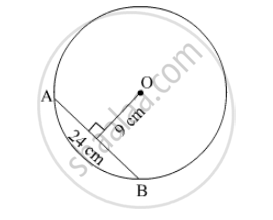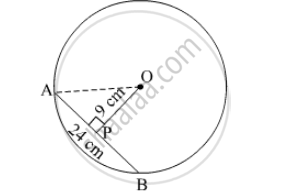# O is Centre of the Circle. Find the Length of Radius, If the Chord of Length 24 Cm is at a Distance of 9 Cm from the Centre of the Circle. - Mathematics

Sum

O is centre of the circle. Find the length of radius, if the chord of length 24 cm is at a distance of 9 cm from the centre of the circle.#### Solution

Join OA.Let the perpendicular drawn from point O to the chord AB be P.
We know that the perpendicular drawn from the centre of the circle to the chord bisects the chord.
So, AP = "AB"/2 = 24/2 = 12 cm

In Δ OPA,
We apply the Pythagoras theorem,

OP² + AP² = OA²

⇒ 9² + 12² = OA²

⇒ OA² = 81 +144 = 225

⇒ OA = √225 15 cm

Hence, the radius of the circle is 15 cm.

Concept: Properties of Chord of a Circle
Is there an error in this question or solution?

#### APPEARS IN

Balbharati Mathematics 8th Standard Maharashtra State Board
Chapter 17 Circle : Chord and Arc
Practice Set 17.1 | Q 3 | Page 116